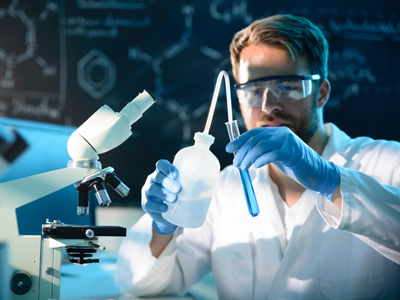If 9g of aluminum reacts with 35.5g of chlorine, the empirical formula of the compound formed is A1C13

# Analysing Substances - Quantitative

This Chemistry quiz is called 'Analysing Substances - Quantitative' and it has been written by teachers to help you if you are studying the subject at high school. Playing educational quizzes is a user-friendly way to learn if you are in the 9th or 10th grade - aged 14 to 16.

It costs only \$12.50 per month to play this quiz and over 3,500 others that help you with your school work. You can subscribe on the page at Join Us

Analyzing substances forms a major part of Chemistry in high school. This quiz is all about quantitative analysis. This kind of analysis allows us to identify the amounts of different elements present in compounds.

Analyzing substances quantitatively answers the question of 'how much'. It involves carrying out experiments that give you results for use in calculations. The calculations indicate the amouts of each element present in a compound and can be given as a percentage or as a mass. These calculations often involve the use of a quantity called the mole. Many students are put off by this as it is not something that they have come across before high school. It should not be worrying - it is just like using more familiar terms like 'thousand', 'million', 'billion' and so on. It is just a word that is used to represent a large number of particles and it really makes life much easier when carrying out chemical calculations.

A mole represents 6.03 x 1023 paricles of a substance, but it doesn't matter if you can't remember that!. The number is so large because atoms and molecules are so tiny.

The main calculations in quantitative analysis depend on your ability to work out the relative formula mass of any given substance. Most of the time you will be given the correct formula but the highest level candidates will show that they can work out the correct formula for a compound from any data given.

The mole simplifies chemical calculations by telling you the proportions of each substance that are involved in a chemical reaction. Look at the following chemical equation:

2H2 + O2 → 2H2O

The numbers in front of each of the formulae indicate the number of moles required. No number in front indicates 1 mole. So in the above reaction, 2 moles of hydrogen molecules react with 1 mole of oxygen molecules to form 2 moles of water molecules - a ratio of 2:1:2.

To work out quantities you only need to know how many moles of ONE of the substances you have to work out the quantities of the rest. If 4 moles of water were produced in the reaction above, you would need 4 moles of hydrogen molecules and 2 of oxygen. If only 0.5 moles of water were produced, you would need 0.5 moles of hydrogen molecules and 0.25 moles of oxygen.

If you need to work out the number of moles for yourself, you use the equation moles = mass/relative formula mass. You will need to rearrange this as required by the questions that you have to answer.

Have a go at this quiz on analyzing substances and see how much you understand about quantitative analysis.

1.
What is the relative formula mass of copper sulfate CuSO4?
160 amu
96 amu
128 amu
144 amu
amu = atomic mass units
2.
What is the relative formula mass of MgF2?
60 amu
62 amu
43 amu
64 amu

Mg = 24 amu, F = 19 amu, remember that there are 2 F atoms in the molecule of MgF2

3.
What % mass of magnesium oxide is magnesium?
60%
40%
30%
70%
First you work out the relative formula mass of magnesium oxide (MgO) then divide the relative atomic mass of magnesium by the relative formula mass and multiply by 100 for the percentage
4.
What is the % mass of hydrogen in methane CH4?
20%
25%
75%
12.5%
Use the same method as for the previous question
5.
Calcium reacts with oxygen as shown by this reaction 2Ca + O2 → 2CaO. What mass of oxygen will react with 60g of calcium?
12g
24g
6g
14g
From the equation, you find that they react in a ratio of 2 moles to 1 mole. 60g of calcium is 1.5 moles so you will need 0.75 moles of oxygen molecules
6.
Sodium hydroxide reacts with chlorine to make bleach as shown by this reaction. 2NaOH + Cl2 → NaOCl + NaCl + H2O. If we have a solution containing 100g of sodium hydroxide, how much chlorine gas should we pass through the solution to make bleach?
28.65g
82.45g
88.75g
86.57g

Ratio of sodium hydroxide to chlorine is 2 moles to1 mole

7.
If 9g of aluminum reacts with 35.5g of chlorine, what is the empirical formula of the compound formed?
AlCl
AlCl2
AlCl3
Al3Cl
Use moles! One third of a mole of aluminum atoms react with 1 mole of chlorine atoms therefore there are three times as many chlorine atoms involved. Remember, the mole is nothing more complicated than a measure of the number of particles in a reaction
8.
A compound contains 13.5g of calcium, 10.8g of oxygen and 0.675g of hydrogen. What is its empirical formula?
CaOH
CaOH2
CaO2H
Ca(OH)2
It's those moles again ...
9.
A reaction that could theoretically produce 200g of product only produces 140g. What is its % yield?
70%
14.2%
142%
7%
Divide the actual yield by the theoretical yield and multiply by 100
10.
Ammonium nitrate NH4NO3 is used as a fertilizer. What is the % mass of nitrogen in it?
21%
37%
17.5%
35%
Divide the total relative atomic mass of the nitrogen in the formula by the relative formula mass and multiply by 100
Author:  Kate Gardiner